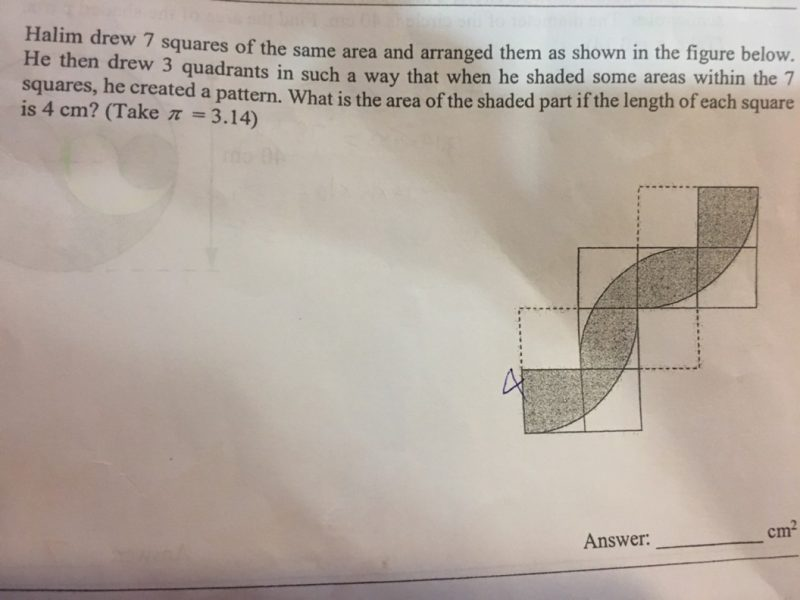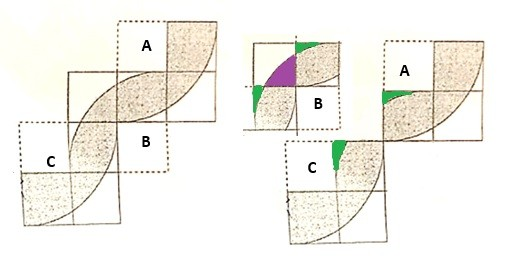QuestionHelp needed

TIAThe diagram can be segregated to 3 parts, A B and C as shown.

Area of shaded portion in A and C

Shaded area = Area of 4 squares – Unshaded area below quadrant – excluded square A (or C)

Area of 4 squares = 4 x 16 = 64cm2

Area of quadrant = (3.14 x 8 x 8) / 4  = 50.24 cm

Unshaded area below quadrant = 64 – 50.24 = 13.76 cm2

Area of excluded square = 16 cm

Shaded area = 64 – 13.76 – 16 = 34.24 cm2

Shaded area in B

Only part filled in purple is useful.

Shaded area = Area of 4 squares – Unshaded area above – 3 squares

Area of 3 squares = 3 x 16 = 48 cm2

Shaded area = 64 – 48 – 13.76 – 16 = 2.24 cm2

Total shaded area = 34.24 + 34.24 + 2.2.4 = 70.72 cm2

The part filled in green, is overlapping and is eliminated for part B, when subtracting the 3 squares. The overlapping part in A and C is eliminated when subtracting the ‘un-shaded area above’ in computation for part B.

0 Replies 0 Likes